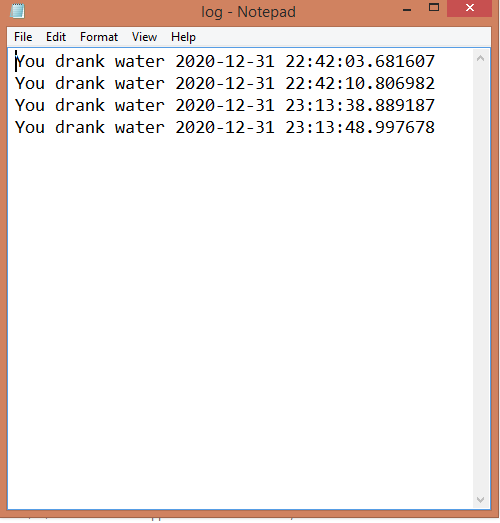# Drink Water notification system in Python

• Last Updated : 13 Jan, 2021

The idea behind this article is to create a notification system that reminds the user to drink water after a fixed interval of time. The program coded below first asks its user to input time interval for notification. Then until user ends script, it sends repeated notifications to user to drink water. After each interval it creates a text file which contains a log of when user drank water.

### Module Used:

• time: To manage the interval time.
• win10Toast : To send quick notification.
• datetime : To keep record of time and date in log.

### Approach

• Import module
• Get time interval from the user, it asks user to input hour, minutes and seconds
• Convert them to seconds and return time to main function.
• Add loop to start timer and generate a toast message when timer reaches the set time
• After sending notification program will call to function to write log file. This function will get current time and date
• Create a .txt file and append drink water log to it.

Program:

## Python3

 `import` `time``from` `win10toast ``import` `ToastNotifier``import` `datetime`` ` ` ` `def` `getTimeInput():``    ``hour ``=` `int``(``input``(``"hours of interval :"``))``    ``minutes ``=` `int``(``input``(``"Mins of interval :"``))``    ``seconds ``=` `int``(``input``(``"Secs of interval :"``))``    ``time_interval ``=` `seconds``+``(minutes``*``60``)``+``(hour``*``3600``)``    ``return` `time_interval`` ` ` ` `def` `log():``    ``now ``=` `datetime.datetime.now()``    ``start_time ``=` `now.strftime(``"%H:%M:%S"``)``    ``with ``open``(``"log.txt"``, ``'a'``) as f:``        ``f.write(f``"You drank water {now} \n"``)``    ``return` `0`` ` ` ` `def` `notify():``    ``notification ``=` `ToastNotifier()``    ``notification.show_toast(``"Time to drink water"``)``    ``log()``    ``return` `0`` ` ` ` `def` `starttime(time_interval):``    ``while` `True``:``        ``time.sleep(time_interval)``        ``notify()`` ` ` ` `if` `__name__ ``=``=` `'__main__'``:``    ``time_interval ``=` `getTimeInput()``    ``starttime(time_interval)`

Output:My Personal Notes arrow_drop_up